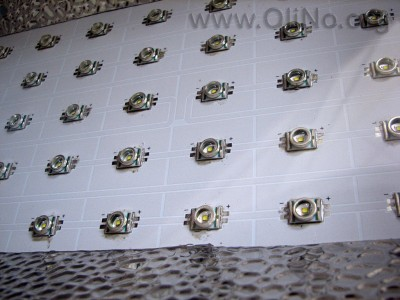# OliNo

Renewable Energy

## Line Lite International BV 150 watt Cree PowerLED Streetlight 50 x 3 watt IP65 60 degr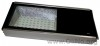presents a streetlight. This has 50 power leds giving a lot of cold white light. This article shows the measurement results. Many parameters are also found in the Eulumdat file.

See this overview for a comparison with other light bulbs.

### Summary measurement data

parameter meas. result remark
Color temperature 8446 K Cold white
Luminous intensity Iv 3063 Cd
Beam angle 87 deg
Power P 135 W
Power Factor 0.73 For every 1 kWh net power consumed, there has been 0.9 kVAhr for reactive power..
Luminous flux 5372 lm
Luminous efficacy 40 lm/W
CRI_Ra 79 Color Rendering Index.
Coordinates chromaticity diagram x=0.2930 and y=0.2957
Fitting 230V Direct connection to the mains voltage.
L x W x H external dimensions 695 x 315 x 130 mm External dimensions of the street light.
L x W luminous area 352 x 215 mm Dimensions of the luminous area (used in Eulumdat file). This is equal to the surface of the transparent part of the glass plate in front of the leds.
form factor street light
General remarks The ambient temperature during the whole set of measurements was 28-28.5 deg C.Warm up effect: during the warm up time the illuminance will decrease about 10 %.

Voltage dependency: the power consumption is a little dependent on the input voltage (in a linear way), the illuminance not at all.

Measurement report (PDF)Eulumdat fileRight click on icon and save the file.

### Eulumdat light diagram

An interesting graph is the light diagram, indicating the intensity in the C0-C180 and the C90-C270 plane. This light diagram below comes from the program Qlumedit, that extracts these diagrams from an Eulumdat file.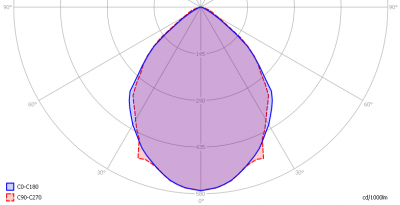The light diagram giving the radiation pattern.

It indicates the luminous intensity around the light bulb. The C0-C180 plane has the same direction as the street over which this light bulb will be placed. The C90-C270 direction is along the length of the light bulb. The C90 plane is situation at the front side of the light bulb (this is where the transparent glass plate hits the side of the housing).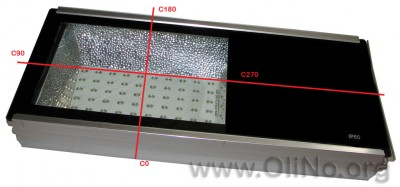The convention used for C-planes for street lights

There is little difference between the diagrams for the given planes.

The unit is Cd/1000lm, meaning the intensity in Cd assuming there would be 1000 lumen in the measured light bulb. This enables comparing different types of light bulbs.

### Illuminance Ev at 1 m distance, or luminous intensity Iv

Herewith the plot of the averaged luminous intensity Iv as a function of the inclination angle with the light bulb.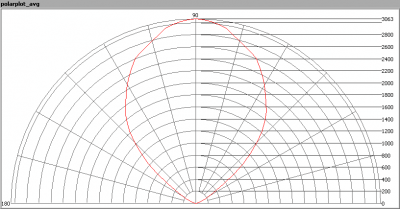The radiation pattern of the light bulb.

This radiation pattern is the average of the light output of the light diagram given earlier. Also, in this graph the luminous intensity is given in Cd.

These averaged values are used (later) to compute the lumen output.Intensity data of every measured turn angle at each inclination angle.

This plot shows per inclination angle the intensity measurement results for each turn angle at that inclination angle. There are differences in illuminance values for different turn angles. However for further calculations the averaged values will be used.

When using the average values per inclination angle, the beam angle can be computed, being 87 degrees. This is the average value for all C-planes on average. There is little difference between the C-planes so this beam angle is accurate for most C-planes.

### Luminous flux

With the averaged illuminance data at 1 m distance, taken from the graph showing the averaged radiation pattern, it is possible to compute the luminous flux.

The result of this computation for this light spot is a luminous flux of 5372 lm.

### Luminous efficacy

The luminous flux being 5372, and the power of the lightbulb being 135 W, yields a luminous efficacy of 40 lm/W.

A power factor of 0.73 means that for every 1 kWh net power consumed, a reactive component of 0.9 kVAr was needed.

 Light bulb voltage (used on power supply!) 230 VAC Light bulb current 805 mA Power P 135 W Apparent power S 185 VA Power factor 0.73

Of this light bulb the voltage across ad the resulting current through it+its power supply are measured and graphed.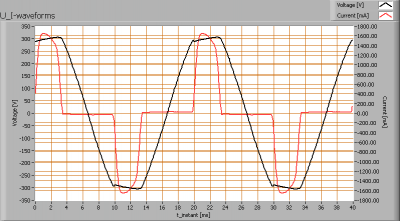Voltage across and current through the lightbulb
There are peaks in current near the tops of the voltage. This results in a waveform for the current that is different from the sineform of the voltage, resulting an a power factor less than 1.

The current drawn in high peaks has as a consequence that the mains voltage tops are capped.

Also the power spectrum of the current is determined.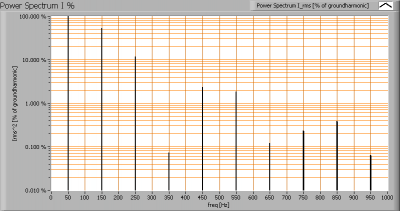Current power spectrum in % of the first harmonic (50 Hz).

The current peaks result in a current power spectrum with a considerable amount of higher harmonics.

### Color temperature and Spectral power distribution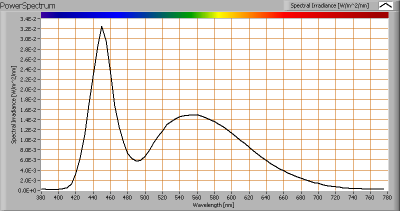The spectral power distribution of this light bulb.

The measured color temperature is about 8500 K which is cold white.

This color temperature is measured straight underneath the light bulb. Below a graph showing the color temperature for different inclination angles.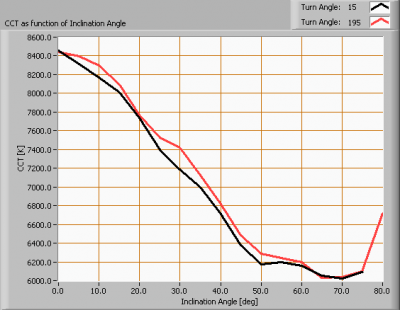Color temperature as a function of inclination angle.

The color temperature is given for inclination angles up to 70 degrees, since at higher angles there is almost no light output anymore.

The value remains constant within 25 %.

### Chromaticity diagram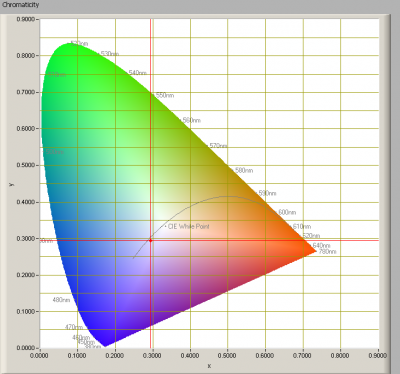The chromaticity space and the position of the lamp’s color coordinates in it.

The light coming from this lamp is at some distance from the Planckian Locus (the black path in the graph).

Its coordinates are x=0.2930 and y=0.2957.

### Color Rendering Index (CRI) or also Ra

Herewith the image showing the CRI as well as how well different colors are represented (rendered). The higher the number, the better the resemblance with the color when a black body radiator would have been used (the sun, or an incandescent lamp).

Each color has an index Rx, and the first 8 indexes (R1 .. R8) are averaged to compute the Ra which is equivalent to the CRI.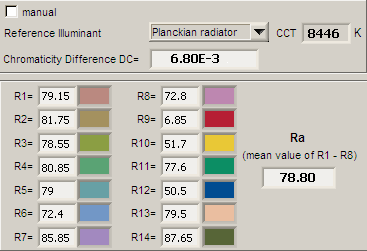CRI of the light of this lightbulb.

The value of 79 is lower than 80 which is considered a minimum value for indoor usage.

Note: the chromaticity difference is 0.0068 indicates the distance to the Planckian Locus. Its value is higher than 0.0054, which means that the calculated CRI result is meaningful. As this led light is not used for indoor usage, it is not of a problem.

### Voltage dependency

The dependency of a number of lamp parameters on the lamp voltage is determined. For this, the lamp voltage has been varied and its effect on the following light bulb parameters measured: illuminance E_v [lx], the lamppower P [W], the (Correlated) Color Temperature [K] and the luminous efficacy [lm/W].Lamp voltage dependencies of certain light bulb parameters, where the value at 230 V is taken as 100 %.

The consumed power is the only parameter that varies (and the luminous efficacy as a consequence), in a linear way, when the voltage varies between 200-250 V. The variation is small.

When the voltage at 230 V varies with + and – 5 V, then the illuminance varies within 0.1 %, so when abrupt voltage changes occur this effect is not visible in the illuminance output.

### Warm up effects

After switch on of a cold lamp, the effect of heating up of the lamp is measured on illuminance E_v [lx], color temperature CT or correlated color temperature CCT [K], the lamppower P [W] and the luminous efficacy [lm/W].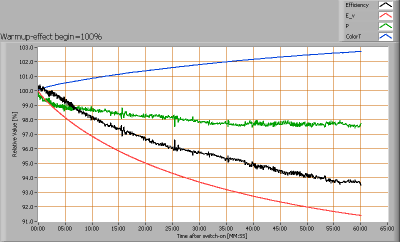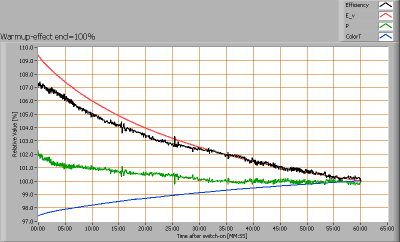Effect of warming up on different light bulb parameters. At top the 100 % level is put at begin, and at bottom at the end.

The warm-up time is a bit more than one hour, and expected illumination drop is about 10 %.